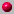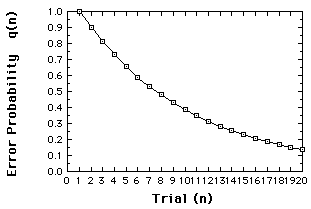## Introduction to Dynamic Systems

### What is a dynamic system?

A dynamic system is a set of functions (rules, equations) that specify how variables change over time.
First example ...
Alice's height diminishes by half every minute...

Second example ...
xnew = xold + yold
ynew= xold

The second example illustrates a system with two variables, x and y. Variable x is changed by taking its old value and adding the current value of y. And y is changed by becoming x's old value. Silly system? Perhaps. We're just showing that a dynamic system is any well-specified set of rules.

#### Here are some important Distinctions:variables (dimensions) vs. parametersdiscrete
vs. continuous variablesstochastic vs. deterministic dynamic systems

How they differ:Variables change in time, parameters do not.Discrete variables are restricted to integer values, continuous variable are not.Stochastic systems are one-to-many; deterministic systems are one-to-one

This last distinction will be made clearer as we go along ...

### Terms

The current state of a dynamic system is specified by the current value of its variables, x, y, z, ...
The process of calculating the new state of a discrete system is called iteration.

To evaluate how a system behaves, we need the functions, parameter values and initial conditions or starting state.
To illustrate...Consider a classic learning theory, the alpha model, which specifies how qn, the probability of making an error on trial n, changed from one trial to the next
qn+1 = ß qn The new error probability is diminished by ß (which is less than 1, greater than 0). For example, let the the probability of an error on trial 1 equal to 1, and ß equal .9. Now we can calculate the dynamics by iterating the function, and plot the results.q1 = 1

q2 = ßq1 = (.9)(1) = .9

q3 = (.9)q2 = (.9)(.9) = .81

etc. ...

Error probabilities for the alpha model, assuming q1=1, ß =.9. This "learning curve" is referred to as a time series.

So far, we have some new ideas, but much is old ...

### What's not new

Dynamic Systems
Certainly the idea that systems change in time is not new. Nor is the idea that the changes are probabilistic.

### What's new

Deterministic nonlinear dynamic systems.

As we will see, these systems give us:

• A new meaning to the term unpredictable.
• A different attitude toward the concept of variability.
• Some new tools for exploring time series data and for modeling such behavior.
• And, some argue, a new paradigm.

This last point is not pursued here.